# Verbal Reasoning - Dice

### Exercise :: Dice - Dice 1

6.

Which number is on the face opposite to 6?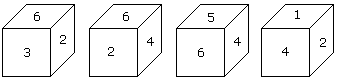A. 4 B. 1 C. 2 D. 3

Explanation:

As the numbers 2, 3, 4 and 5 are adjacent to 6. Hence the number on the face opposite to 6 is 1.

7.

Two positions of a dice are shown below. When number '1' is on the top. What number will be at the bottom?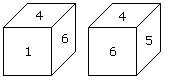A. 3 B. 5 C. 2 D. 6

Explanation:

According to the rule (2) when 'one' is at the top, then 5 will be at the bottom.

8.

Two positions of a cube with its surfaces numbered are shown below. When the surface 4 touch the bottom, what surface will be on the top?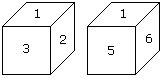A. 1 B. 2 C. 5 D. 6

Explanation:

In these 2 positions one common face with number 1 is in the same position. Hence according to the rule number (3), 2 is opposite 6 and 3 is opposite to 5. Therefore opposite to 4 is 1.

9.

Here two positions of dice are shown. If there are two dots in the bottom, then how many dots will be on the top?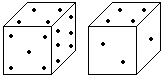A. 2 B. 3 C. 5 D. 6

Explanation:

Here the common faces with 4 dots are in same positions.

Hence 2 will be opposite to 5.

10.

Two positions of dice are shown below. How many points will be on the top when 2 points are at the bottom?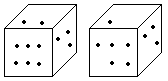A. 6 B. 5 C. 4 D. 1